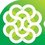# Discuss more about: Pyramid Investigations by Arron Kau

After solving all 6 problems, I just remembered a very fast, easy and awesome way to solve other problems like these 6 problems. (Note: You can easily prove my equations!)

Back to our easy equations:

$1={ 1 }^{ 2 }$
$1+2+1={ 2 }^{ 2 }$

$1+2+3+2+1={ 3 }^{ 2 }$
...

And: $1={ 1 }$
$1+2=\frac { 2\times (2+1) }{ 2 }$
$1+2+3=\frac { 3\times (3+1) }{ 2 }$
...

These are very simple right, I think you can prove them easily. But there is a question: Can you calculate quickly this sum: $1 + 4 + 7 + ... + 34 = ?$

My answer is: Yes, you can! But how?

Using this sum above First, calculate the total terms of this sum: Numbers of Terms $= \frac { ( \text{ The last term - The first term} ) } { \text{ The difference between each term}} +1$.
In this equation, the number of terms is: $\frac { 34-1 }{ 3 } +1=12$

Our first step is completed, now move to the next step To sum this equation, use this: The sum $= \frac {{ ( \text{The first term + The last term}) \times} {\text {Number of terms }}}{2}$

So in this equation: The sum is: $\frac { (34+1)\times 12 }{ 2 } =210$

This is my solution, and you can use it in all arithmetic series. I hope to discuss more with all of you.Note by Dang Anh Tu
7 years, 2 months ago

This discussion board is a place to discuss our Daily Challenges and the math and science related to those challenges. Explanations are more than just a solution — they should explain the steps and thinking strategies that you used to obtain the solution. Comments should further the discussion of math and science.

When posting on Brilliant:

• Use the emojis to react to an explanation, whether you're congratulating a job well done , or just really confused .
• Ask specific questions about the challenge or the steps in somebody's explanation. Well-posed questions can add a lot to the discussion, but posting "I don't understand!" doesn't help anyone.
• Try to contribute something new to the discussion, whether it is an extension, generalization or other idea related to the challenge.
• Stay on topic — we're all here to learn more about math and science, not to hear about your favorite get-rich-quick scheme or current world events.

MarkdownAppears as
*italics* or _italics_ italics
**bold** or __bold__ bold
- bulleted- list
• bulleted
• list
1. numbered2. list
1. numbered
2. list
Note: you must add a full line of space before and after lists for them to show up correctly
paragraph 1paragraph 2

paragraph 1

paragraph 2

[example link](https://brilliant.org)example link
> This is a quote
This is a quote
    # I indented these lines
# 4 spaces, and now they show
# up as a code block.

print "hello world"
# I indented these lines
# 4 spaces, and now they show
# up as a code block.

print "hello world"
MathAppears as
Remember to wrap math in $$ ... $$ or $ ... $ to ensure proper formatting.
2 \times 3 $2 \times 3$
2^{34} $2^{34}$
a_{i-1} $a_{i-1}$
\frac{2}{3} $\frac{2}{3}$
\sqrt{2} $\sqrt{2}$
\sum_{i=1}^3 $\sum_{i=1}^3$
\sin \theta $\sin \theta$
\boxed{123} $\boxed{123}$

## Comments

Sort by:

Top Newest

Note: To use latex, you have to place it within the brackets \ ( code \ ), with spaces removed. Also, you don't need to use \quad to space out text. Just leave them outside of the brackets. If you want to include text, I would suggest using \text{ XXX } instead, as this makes it display as per normal, instead of italicised.

I edited the first few paragraphs of your note, to give you an example. You can edit it to see the changes that I made.

Staff - 7 years, 2 months ago

Log in to reply

Oh, thank you. I am just a newbie here!

- 7 years, 2 months ago

Log in to reply

Looks great! You are a fast learner.

Staff - 7 years, 2 months ago

Log in to reply

Thank you for your help!

- 7 years, 2 months ago

Log in to reply

×

Problem Loading...

Note Loading...

Set Loading...